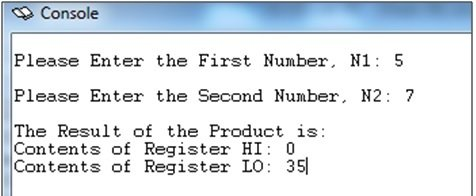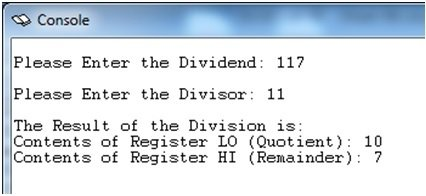# Multiplication and Division in MIPS Assembly Language

### Integer Multiplication in the MIPS Assembly Language

The generic form of the mult (signed integer multiplication) and multu (unsigned integer multiplication) instructions is:

Signed Integer Multiplication:              mult  \$Rs, \$Rt

Unsigned Integer Multiplication:          multu \$Rs, \$Rt

mult and multu belong to the Arithmetic Core Instruction Set and are R-type instructions where \$Rd, the destination register, does not appear. So where does the result go?  The result automatically goes into two special registers called HI and LO.

The semantics are given below:

(HI, LO) = Rs * Rt

We know that multiplying the contents of two 32-bit registers will give a 64-bit result. The high 32 bits are placed in a register called HI. The low 32 bits are placed in a register called LO. You only need to read LO if you know your result fits into the Lower 32-bits.

To access the HI and LO registers, which are 2 additional registers beyond the 32 integer registers and are specifically used for integer multiplication and division, we use the following instructions:

mfhi  \$Rd                    for example, mfhi \$a0

mflo  \$Rd

mfhi means “move from HI” to the destination register. mflo means “move from LO” to the destination register.

Exercise-1: Write a code that reads two numbers and returns their product:

Your program should ask the user to enter two integer numbers at the PCSPIM console window. It should read these numbers and perform their integer multiplication. The result of the product should also be printed at the console of PCSPIM. For now, perform this multiplication for such numbers that the result is accommodated in 32-bits and thus fits into LO. However, print the contents of both HI and LO on the console. We have given the .data segment of the code below. Fill in the blanks in the data segment and develop your code in the text segment accordingly.

Code:

```.data

Number1: .asciiz "\nPlease Enter the First Number, N1: "

Number2: .asciiz "\nPlease Enter the Second Number, N2: "

Result:   .asciiz "\nThe Result of the Product is: "

High:     .asciiz "\nContents of Register HI: "

Low:      .asciiz "\nContents of Register LO: "

.text

.globl main

main: (Write the rest of the code below)

li \$v0, 4

la \$a0, Number1

syscall

li \$v0, 5

syscall

li \$v0, 4

la \$a0, Number2

syscall

li \$v0, 5

syscall

mult \$t0, \$t1

li \$v0, 4

la \$a0, Result

syscall

li \$v0, 4

la \$a0, High

syscall

li \$v0, 1

mfhi \$a0

syscall

li \$v0, 4

la \$a0, Low

syscall

li \$v0, 1

mflo \$a0

syscall

End_Prog:

li \$v0, 10

syscall```

Figure 1: Example of how the console window should look like after the execution of your program in Exercise-1### Integer Division in the MIPS Assembly Language

The generic form of the div (signed integer division) and divu (unsigned integer division) instructions is:

Signed Integer Division:                       div  \$Rs, \$Rt

Unsigned Integer Division:                 divu \$Rs, \$Rt

div and divu belong to the Arithmetic Core Instruction Set and are R-type instructions where \$Rd, the destination register, does not appear. The registers HI and LO are used here as well

The semantics are given below:

LO = Quotient of (Rs ÷ Rt)

HI = Remainder of (Rs ÷ Rt)

As in Task-1, the instructions mfhi and mflo will be used to copy the contents of the registers HI and LO respectively into some destination register Rd.

mfhi  \$Rd                   for example, mfhi \$a0

mflo  \$Rd

mfhi means “move from HI” to the destination register. mflo means “move from LO” to the destination register.

Exercise-2: Write a code that reads two numbers and performs division:

Your program should ask the user to input two integer numbers at the PCSPIM console window, one of them being the dividend and the other being the divisor. It should then perform the necessary division. The result of the division should also be printed at the console of PCSPIM in terms of Quotient and Remainder. We have given the .data segment of the code below. Fill in the blanks in the data segment and develop your code in the text segment accordingly.

Code:

```.data

Dividend:     .asciiz "\nPlease Enter the Dividend: "

Divisor:      .asciiz "\nPlease Enter the Divisor: "

Result:       .asciiz "\nThe Result of the Division is: "

Quotient:     .asciiz "\nContents of Register LO (Quotient): "

Remainder:    .asciiz "\nContents of Register HI (Remainder): "

.text

.globl main

main: #(Code not required in the actual Lab-5 Submission)

li \$v0, 4

la \$a0, Dividend

syscall

li \$v0, 5

syscall

li \$v0, 4

la \$a0, Divisor

syscall

li \$v0, 5

syscall

div \$t0, \$t1

li \$v0, 4

la \$a0, Result

syscall

li \$v0, 4

la \$a0, Quotient

syscall

li \$v0, 1

mflo \$a0

syscall

li \$v0, 4

la \$a0, Remainder

syscall

li \$v0, 1

mfhi \$a0

syscall

End_Prog:

li \$v0, 10

syscall```

Figure 2: Example of how the console window should look like after the execution of your program in Exercise-2